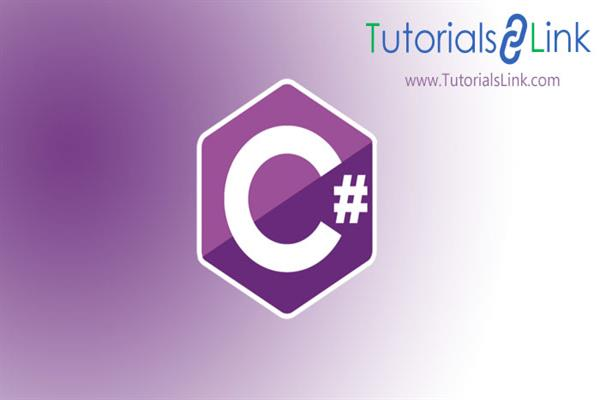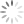Loading, please wait...# Arrays MCQs (Multiple Choice Questions and Answers) in C#

May 29, 2020 C#, CSharp, Arrays, ArraysInC#, McQS, 47982 Views

## ARRAYS in C# - MCQs

### Q.1 Which of the following statements is correct about the C#.NET code snippet given below?

``int[] intMyArr = {11, 3, 5, 9, 4};``

Options:

1.     intMyArr is a reference to an object of System.Array Class.
2.     intMyArr is a reference to an object of a class that the compiler derives from the System.Array Class.
3.     intMyArr is a reference to an array of integers.
4.     intMyArr is a reference to an object created on the stack.

### Q.2 Which of the following is the correct way to define and initialize an array of 4 integers?

1.

``int[] a = {25, 30, 40, 5};``

2.

``````int[] a;
a = new int;
a = 25;
a = 30;
a = 40;
a = 5;``````

3.

``````int[] a;
a = new int{25, 30, 40, 5};``````

4.

``````int[] a;
a = new int{25, 30, 40, 5};``````

5.

``````int[] a;
a = new int;
a = 25;
a = 30;
a = 40;
a = 5;``````

Options:

1. 1, 2
2. 3, 4
3. 1, 4, 5
4. 2, 4, 5
5. 2, 5

### Q.3. Which of the following is the correct output of the C#.NET code snippet given below?

``````int[][] a = new int[];
a = new int{6, 1, 4, 3};
a = new int{9, 2, 7};
Console.WriteLine(a.GetUpperBound(0));``````

Options:

1. 3
2. 4
3. 7
4. 2

### Q.4. Which of the following is the correct way to obtain the number of elements present in the array given below?

int[] intMyArr = {25, 30, 45, 15, 60};

1.

``intMyArr.GetMax;``

2.

``intMyArr.Highest(0);``

3.

``intMyArr.GetUpperBound(0);​``

4.

``intMyArr.Length;``

5.

``intMyArr.GetMaxElements(0);​``

Options:

1. 1, 2
2. 3, 4
3. 3, 5
4. 1, 5

### Q.5.What will be the output of the C#.NET code snippet given below?

``````namespace ProgramConsoleApplication
{
class SampleProgram
{
static void Main(string[ ] args)
{
int i, j;
int[ , ] arr = new int[ 2, 2 ];
for(i = 0; i < 2; ++i)
{
for(j = 0; j < 2; ++j)
{
arr[i, j] = i * 17 + i * 17;
Console.Write(arr[ i, j ] + " ");
}
}
}
}
}``````

Options:

1. 0 0 34 34
2. 0 0 17 17
3. 0 0 0 0
4. 17 17 0 0

### Q.6. Which of the following is the correct output of the C#.NET code snippet given below?

``````int[ , , ] a = new int[ 3, 2, 3 ];
Console.WriteLine(a.Length);
``````

Options:

1. 20
2. 4
3. 18
4. 10

### Q.7. Which of the following statements are correct about arrays used in C#.NET?

1. Arrays can be rectangular or jagged.
2. Rectangular arrays have similar rows stored in adjacent memory locations.
3. Jagged arrays do not have access to the methods of System.Array Class.
4. Rectangular arrays do not have access to the methods of System.Array Class.
5. Jagged arrays have dissimilar rows stored in non-adjacent memory locations.

Options:

1. 1, 2
2. 1, 3, 5
3. 3, 4
4. 1, 2, 5

### Q.8. Which of the following statements are correct about the C#.NET code snippet given below?

``````int[][]intMyArr = new int[];
intMyArr = new int{6, 1, 4, 3};
intMyArr = new int{9, 2, 7};``````

Options:

1. intMyArr is a reference to a 2-D jagged array.
2. The two rows of the jagged array intMyArr are stored in adjacent memory locations.
3. intMyArr refers to the zeroth 1-D array and intMyArr refers to the first 1-D array.
4. intMyArr refers to intMyArr and intMyArr.

### Q.9. Which of the following are the correct ways to define an array of 2 rows and 3 columns?

1.

``````int[ , ] a;
a = new int[2, 3]{{7, 1, 3},{2, 9, 6}};``````

2.

``````int[ , ] a;
a = new int[2, 3]{};``````

3.

``int[ , ] a = {{7, 1, 3}, {2, 9,6 }};``

4.

``````int[ , ] a;
a = new int[1, 2];``````

5.

``````int[ , ] a;
a = new int[1, 2]{{7, 1, 3}, {2, 9, 6}};``````

Options:

1. 1, 2, 3
2. 1, 3
3. 2, 3
4. 2, 4, 5

### Q.10. Which of the following statements is correct about the array declaration given below?

``int[][][] intMyArr = new int[][];``

Options:

1. intMyArr refers to a 2-D jagged array containing 2 rows.
2. intMyArr refers to a 2-D jagged array containing 3 rows.
3. intMyArr refers to a 3-D jagged array containing 2 2-D jagged arrays.
4. intMyArr refers to a 3-D jagged array containing three 2-D jagged arrays.

### Q.11. Which of the following statements are correct about the C#.NET code snippet given below?

``int[ , ] intMyArr = {{7, 1, 3}, {2, 9, 6}};``
1. intMyArr represents a rectangular array of 2 rows and 3 columns.
2. intMyArr.GetUpperBound(1) will yield 2.
3. intMyArr.Length will yield 24.
4. intMyArr represents 1-D array of 5 integers.
5. intMyArr.GetUpperBound(0) will yield 2.

Options:

1. 1, 2
2. 2, 3
3. 2, 5
4. 1, 4

### Q.12. Which of the following statements are correct about the C#.NET code snippet given below?

``int[] a = {11, 3, 5, 9, 4};``
1. The array elements are created on the stack.
2. Reference is created on the stack.
3. The array elements are created on the heap.
4. On declaring the array a new array class is created which is derived from System.Array Class.
5. Whether the array elements are stored in the stack or heap depends upon the size of the array.

Options:

1. 1, 2
2. 2, 3, 4
3. 2, 3, 5
4. 4, 5

### Q.13. Which one of the following statements is correct?

1. Array elements can be of integer type only.
2. The rank of an Array is the total number of elements it can contain.
3. The length of an Array is the number of dimensions in the Array.
4. The default value of numeric array elements is zero.

### Q.14. If a is an array of 5 integers then which of the following is the correct way to increase its size to 10 elements?

Options:

1.

``````int[] a = new int;
int[] a = new int;``````

2.

``````int[] a = int;
int[] a = int;``````

3.

``````int[] a = new int;
a.Length = 10 ;``````

4.

``````int[] a = new int;
a = new int;``````

### Q.15. How will you complete the foreach loop in the C#.NET code snippet given below such that it correctly prints all elements of the array a?

``````int[][]a = new int[];
a = new int{6, 1 ,4, 3};
a = new int{9, 2, 7};
foreach (int[ ] i in a)
{
Console.Write(j + " ");
Console.WriteLine();
}``````

Options:

1. foreach (int j = 1; j < a(0).GetUpperBound; j++)
2. foreach (int j = 1; j < a.GetUpperBound (0); j++)
3. foreach (int j in a.Length)
4. foreach (int j in i)

### Q.16. What will be the output of the following C# code?

``````static void Main(string[] args)
{
int i, j;
int[, ] arr = new int[ 3, 3];
for (i = 0; i < 3; ++i)
{
for (j = 0; j < 3; ++j)
{
arr[i, j] = i * 2 + i * 2;
Console.WriteLine(arr[i, j]);
}
}
}``````

Options:

1. ) 0, 0, 0 4, 4, 4  8, 8, 8
2. ) 4, 4, 4 8, 8, 8   12, 12, 12
3. ) .8, 8, 8  12, 12, 12  16, 16, 16
4. )0, 0, 0 1, 1, 1 2, 2, 2

### Q.17. What will be the output of the following C# code?

`````` static void Main(string[] args)
{
char A = 'K';
char B = Convert.ToChar(76);
A++;
B++;
Console.WriteLine(A+ "  " +B);
}``````

Options:

1. M L
2. U L
3. L M
4. A B

### Q.18. Complete the following C# code with “foreach condition”.

``````int[][]a = new int[];
a = new int{3, 4, 2};
a = new int{8, 5};
foreach( int[]i in a)
{
console.write( j+ " ");
console.writeline();
}``````

Options:

1. foreach (int j = 1;(j(<)(a(0).GetUpperBound)); (j++));
2. foreach (int j = 1;(j(<)(a.GetUpperBound(0))); (j++));
3. foreach (int j in a.Length);
4. foreach (int j in i);

### Q.19. What will be the output of the following C# code?

``````static void Main(string[] args)
{
double a = 345.09;
byte c = (byte) a;
Console.WriteLine(c);
}``````

Options:

1. 98
2. 89
3. 88
4. 84

### Q.20. Which statement is correct about following c# .NET code?

``int[] a= {11, 3, 5, 9, 6};``

Options:

1. ‘a’ is a reference to the array created on the stack
2. ‘a’ is a reference to an object created on the stack
3. ‘a’ is a reference to an object of a class that compiler drives from ‘System.Array’ class
4. None of the mentioned

### Q.21. What is the advantage of using 2D jagged array over 2D rectangular array?

Options:

1. Easy initialization of elements
2. Allows unlimited elements as well as rows which had ‘0’ or are empty in nature
3. All of the mentioned
4. None of the mentioned

### Q.22. Which statement is correct about following C# code?

``int[, ]a={{5, 4, 3},{9, 2, 6}};``

Options:

1. ’a’ represents 1-D array of 5 integers
2. a.GetUpperBound(0) gives 9
3. ’a’ represents a rectangular array of 2 columns and 3 arrays
4. a.GetUpperBound(0) gives 2

### Q.23. What will be the output of the following C# code?

``````static void Main(string[] args)
{
Program p = new Program();
p.display(2, 3, 8);
int []a = { 2, 56, 78, 66 };
Console.WriteLine("example of array");
p.display(a);
}
public void display(params int[] b)
{
foreach (int i in b)
{
Console.WriteLine("ARRAY IS HAVING:{0}", i);
}
}``````

Options:

1.     Compile-time error
2.     Run time error
3.     Code runs successfully but prints nothing
4.     Code runs successfully and prints gave on console

### Q.24. Which is the correct way of defining and initializing an array of 3 integers?

Options:

1.

``int[] a={78, 54};``

2.

``````int[] a;
a = new int;
a = 78;
a = 9;
a = 54;``````

3.

``````int[] a;
a = new int{78, 9, 54};``````

4.

``````int[] a;
a = new int{78, 9, 54};``````